# Oscillations and Waves   Share

## What is Oscillations and Waves

The most basic examples of oscillation are rocking in a cradle and swinging on a swing. If you will think critically then you will realize that in both of these examples the motion is repetitive and the swing or cradle goes to and fro about a specific point. Another example is the pendulum wall clock, in which the pendulum repeats its motion periodically. If many objects come together and do oscillatory motion then they form a wave. In order to understand the former statement consider the disturbance created in a pond when you drop a stone in the still water. It will seem like the disturbance (ripples) is moving outwards in the circular form. But if you will place cork on the disturbance you will see that the cork is not moving outwards it will be just oscillating where you placed it. The disturbance is a form of a wave in which the energy is transferred from one particle to another rather than actual physical transfer.## Oscillations and Waves- Topics

Oscillation: Periodic motion: period, frequency and displacement as a function of time. Periodic functions. Simple harmonic motion, equation of SHM, phase. Oscillation of spring, spring constant and restoring force. Energies in SHM: kinetic and potential energies. Simple pendulum, derivation of the time period of a simple pendulum. Free, forced, and damped oscillations, resonance

Waves: Longitudinal and transverse wave, wave speed, displacement relation for progressive wave, superposition and reflection of waves. Standing waves in the string and organ pipes, harmonics and fundamental mode, beats, dopplers effect

## Overview of Oscillations and Waves

Oscillatory motion is a type of periodic motion but in this motion, the object moves to and fro about the mean position in regular interval of time. It is important to understand that every oscillatory motion is periodic motion but every periodic motion is not oscillatory. For example, the rotation of the earth around the sun is periodic but not oscillatory. In this chapter, you will mainly have to read about Simple Harmonic Motion (SHM). SHM is the simplest form of oscillatory motion in which the restoring force is directly proportional to the displacement of the particle. Where Retarding force is a force which acts on the particle or object to bring it back to its rest position. Now let's understand some basic terms related to Oscillatory Motion :

Prepare Online for JEE Main/NEET

Crack JEE 2021 with JEE/NEET Online Preparation Program

1. Time Period - The smallest interval of time after which the motion starts to repeat itself is known as the time period. It is denoted by T.

2. Frequency - It represents the number of repetition of motion per unit time. In other words, it is reciprocal of the time period. It is denoted by f.

3. Periodic Function - A function which could be expressed by the superposition of sine and cosine functions of different time periods and suitable coefficients.

4. Amplitude - It is a positive constant which represents the maximum displacement of the particle in either direction. It is denoted by A.

5. Phase constant - A phase constant is the initial position/phase of a particle in SHM. It is denoted by.

A wave, on the other hand, consists of the oscillatory motion of the various particle and gradually these oscillations of particles propagate to other points without the actual transport of matter. Equation of waves can also be described by the terms which are mentioned above.

A wave can be classified in three manners :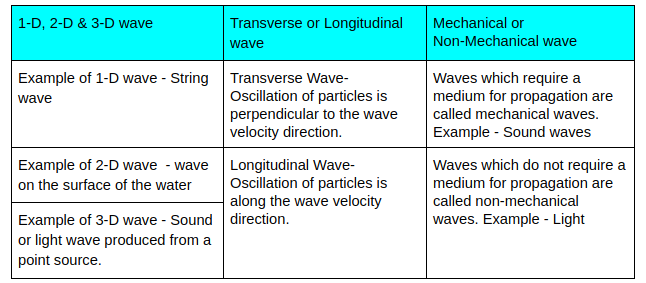## Formulas for Oscillations & Waves

Oscillations

•• Time period for spring oscillator,• Time period for simple pendulum,Waves

• General Equation of sine wave -• Phase Difference,• Wave speed,## How to prepare Oscillations & Waves

When solving questions for both these topics always keep in mind that your concepts should be clear. Most of the questions in this chapter won’t require long calculation, rather they will be concept based. Hence the questions from these chapters are easy but conceptual. You could master both these chapters by practicing more. If you face any problem in solving the question read the topic again and solve. Give mock tests to build confidence. In oscillations and waves, you will find many equations so get familiar with these equations and understand the meaning of each term in the equation.

## Notes for Oscillation and Waves

• Frequently revise your notes, and make a list of weak topics and practice more on that.

• You should be able to convert one entity to another, example - converting time period into angular frequency. Don’t get confused about angular frequency and frequency.

• Give more attention to Standing waves in organ pipe and string, Doppler’s effect, and resonance.

• Clear your doubts with your teacher or classmates or authenticated online resources.

• Always write down what is given in the question.

## Books - Oscillation and Waves

For questions related to oscillations and waves you should, first of all, go through NCERT with giving attention to examples. Try to solve each and every question of NCERT question. For getting more insights about the chapter, solve questions from NCERT Exemplar. But apart from these books, you should also give topic-wise online mock tests. We will provide you with chapter articles of all the chapters, in simple language to make learning easy and a variety of questions in mock tests.

## Physics Chapter wise Notes For Engineering and Medical Exams

 Chapters No. Chapters Name Chapter 1 Physics and Measurement Chapter 2 Kinematics Chapter 3 Laws of motion Chapter 4 Work Energy and Power Chapter 5 Rotational Motion Chapter 6 Gravitation Chapter 7 Properties of Solids and Liquids Chapter 8 Kinetic theory of Gases Chapter 9 Thermodynamics Chapter 11 Electrostatics Chapter 12 Current Electricity Chapter 13 Magnetic Effects of Current and Magnetism Chapter 14 Electromagnetic Induction and Alternating currents Chapter 15 Electromagnetic Waves Chapter 16 Optics Chapter 17 Dual Nature of Matter and Radiation Chapter  18 Atoms And Nuclei Chapter 19 Electronic devices Chapter 20 Communication Systems Chapter 21 Experimental skills

### Topics from Oscillations and Waves

• Periodic motion: period, frequency, displacement as a function of time ( JEE Main, COMEDK UGET, KEAM ) (4 concepts)
• Simple harmonic motion (S.H.M.) and its equation; phase ( JEE Main, COMEDK UGET, KEAM ) (18 concepts)
• energy in S.H.M. kinetic and potential energies ( JEE Main, COMEDK UGET, KEAM ) (6 concepts)
• oscillations of a spring -restoring force and force constant ( JEE Main, COMEDK UGET, KEAM ) (5 concepts)
• Simple pendulum derivation of expression for its time period ( JEE Main, COMEDK UGET, KEAM ) (13 concepts)
• Free, forced and damped oscillations, resonance. ( JEE Main, COMEDK UGET, KEAM ) (6 concepts)
• Wave motion: Longitudinal and transverse waves ( JEE Main, COMEDK UGET, KEAM ) (8 concepts)
• the speed of a wave. Displacement relation for a progressive wave. ( JEE Main, COMEDK UGET, KEAM ) (31 concepts)
• Beats, Doppler effect in sound. ( JEE Main, COMEDK UGET, KEAM ) (11 concepts)
• The principle of superposition of waves, the reflection of waves, ( JEE Main, COMEDK UGET, KEAM ) (7 concepts)
• Standing waves in strings and organ pipes, fundamental mode and harmonics ( JEE Main, COMEDK UGET, KEAM ) (11 concepts)
• Simple harmonic motion ( SHM ) and its equation ( JEE Main, COMEDK UGET, KEAM ) (1 concepts)
• Oscillation in a spring ( JEE Main, COMEDK UGET, KEAM ) (3 concepts)
• Sound Wave ( JEE Main, COMEDK UGET, KEAM ) (13 concepts)
• waves on a string ( JEE Main, COMEDK UGET, KEAM ) (15 concepts)
• Pendulum and its time period ( JEE Main, COMEDK UGET, KEAM ) (9 concepts)
• Simple harmonic motion ( SHM ) ( JEE Main, COMEDK UGET, KEAM ) (8 concepts)
• Damped and forced oscillations ( JEE Main, COMEDK UGET, KEAM ) (2 concepts)

### Important Books for Oscillations and Waves

•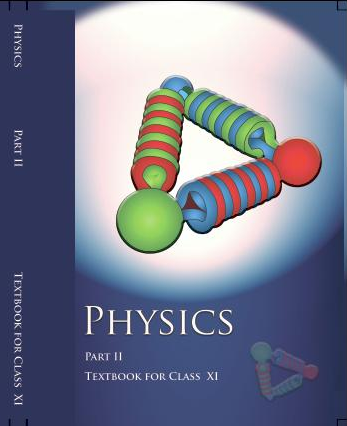•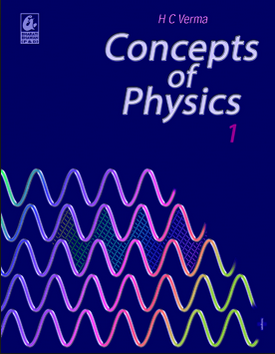•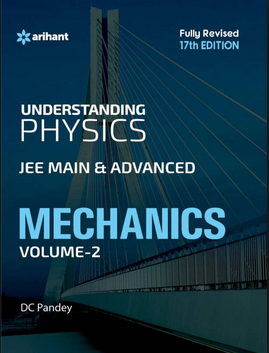•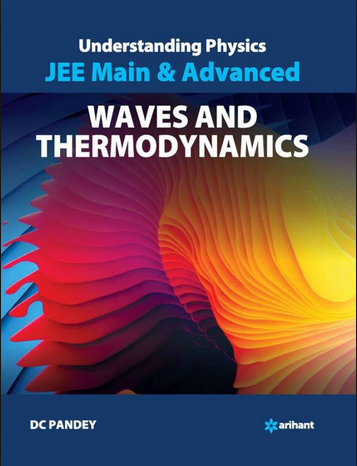Exams
Articles
Questions